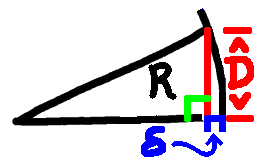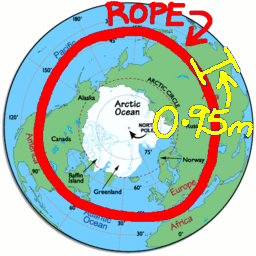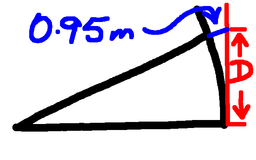# Other Other Other Rope Around The EarthSubscribe!My latest posts can be found here:
Previous blog posts:

## 2019/01/29 - The $(Other)^3$ Rope Around the Earth

So we have:

You might think that was the end of it, but no, there is more!

(I can hear some of you groaning from here)

 My thanks (for some definition of that word) to Alun Jones for this problem.
So what is the Other Other Other Rope around the Earth problem?

Our protagonist again has too much to drink and again gets involved with internet shopping, and again orders 40 million metres of rope to girdle the Earth. But this time, upon travelling all the way around the Earth, trailing the rope behind, it's six metres too short!

Argh!

OK. It won't stretch, we could cut six metres off the other rope, but that's now a tourist attraction, so we need a different solution.

Aha! Let's move it slightly to the North, to a slightly higher latitude, and run it around one of the parallels of latitude. It will still be a circle, but it won't be a Great Circle.

We can picture this as joining the ends of the rope to make a loop, and then throwing it over the Earth. It's too small to fall off, so it gets stuck part way down.

 We are, of course, once again, in "Puzzle World", and pretending that the Earth is a perfect sphere of circumference 40 million metres
How far? How far north of the Equator will it get stuck? What should our latitude be to get a circumference that's six metres shorter than a GREAT Circle?

We can go about this in the obvious way, writing down the formula and using algebra to solve it. It's powerful, and we can solve most things like that. But there is no insight, no connection to other problems.

## The straight-forward algebra version

So we need to know the circumference at a given latitude. That's not hard. At latitude $\theta$ we can picture the circle, and it has a radius of $\cos(\theta)$. So we want $C\cos(\theta)+6=C$, where $C$ is the Earth's circumference.

Rearrange and we have:

• $\cos(\theta)=(C-6)/C$

We know that when $\theta$ is small enough, $\cos(\theta)\approx 1-\theta^2/2$, so we get:

• $1-\theta^2/2 \approx 1-\frac{6}{C}$

So

• $\theta^2/2\approx\frac{6}{C}$

Hence $\theta$ is roughly $\sqrt{12/C}$

Based on that, our actual distance north of the Equator is given by $R\sqrt{12/C}$. But $C=2\pi R$, so simplifying a little that can be re-written as:

• $D\approx\sqrt{(6/\pi)R}$

## But wait - there's more!

In truth, we can do this in a completely straight-forward manner, and it's really not that hard.Pythagoras. Again.
We approximate the distance we want as being the height $D$ of this right-angled triangle, and we know that the "short fall" of the radius is $6/(2\pi)$, which is about 0.95 metres. Writing that as $\delta$ we have:

• $D^2+(R-\delta)^2=R^2$

Expanding, ignoring the $\delta^2$ term, we get:

• $D\approx\sqrt{2\delta R}$

But $\delta=6/(2\pi)$, so we can substitute that in:

• $D\approx\sqrt{2\cdot 6/(2\pi) R}$

which simplifies to:

• $D\approx\sqrt{(6/\pi) R}$

Satisfying that we get the same answer

Approximating $6/\pi$ by 2, and ignoring the "million", we want the square root of 12.8. But $(3.5)^2$ is 12.25, so the answer is about 3.5.

But wait! 12.8 is about 5% bigger than 12.25, and $\pi$ is about 5% bigger than 3, we're multiplying by one, dividing by the other, so these two errors compensate.

## More elegantly ...

So is there a more elegant way to do this? (Of course there is, otherwise I wouldn't've asked ...)

I was struggling with this, then over lunch Rogerio Martins made a comment that provided the necessary insight to be able to solve this neatly, cleanly, and without long, complex calculations.

So how does that work?From Above
There are two steps here. The first is to look at the situation from above, in projection. From this point of view we can see the Equator as the circumference, 40 million metres around. Just inside that we see our rope. It's 6 metres short, so it's 0.95 metres inside, as we computed in The Rope Around The Earth Refined.

Keeping that in mind, we switch to a side view. Now we see the rope some distance above the equator, and ask what that distance is.From the side
Zooming in on the side, we can see that rising just 0.95 metres above the surface of the Earth where the rope lies takes us to the tangent that meets the Earth at the Equator. Saying that in a different way, the distance we want is the distance to the horizon when we are 0.95 metres above the surface of the Earth.

But we have enough information to do that! (Well, I have - and so will you if you've been following along in this series.)

When we are 5 metres up, the distance to the horizon is 8000 metres. It goes as a square root, so we halve the distance by being only a quarter as high, so from 1.25 metres we can see 4000 metres. Not quite what we want, so do it again ... from 0.31 metres (roughly) we can see 2000 metres (roughly).

Now we cheat a little and say that 0.31 is about 1/3 of 0.95, so we are increasing our height by a factor of three, and that increases our distance by a factor of $\sqrt{3}$.

 And the latitude? 3500 metres is about 200 metres short of 2 Nautical Miles, so this distance is a touch short of 2 arc minutes.
So the distance is about 2000 times 1.732, or 3464 metres.

And again, we've done that without calculators, tables, computers, or any aids.

That gives me more pleasure than perhaps it should.

You might like to do the sums on a calculator and see how close these results are, and where the errors are worst.

Can you do better? Let me know ...

## Acknowledgements

My thanks to Alun Jones (Twitter, Web site) for suggesting the problem, Rogerio Martins (Web site) for his insight that led to the solution, and Adam Atkinson and Pedro Freitas for feedback, comments, and corrections.

 <<<< Prev <<<< Rope Around The Earth Refined : >>>> Next >>>> Rounding Up The Ropes ...You can follow me on Mathstodon.

 Of course, you can alsofollow me on twitter:## Send us a comment ...

 You can send us a message here. It doesn't get published, it just sends us an email, and is an easy way to ask any questions, or make any comments, without having to send a separate email. So just fill in the boxes and then

 Your name : Email : Message :

# ContentsSuggest a change ( <-- What does this mean?) / Send me email